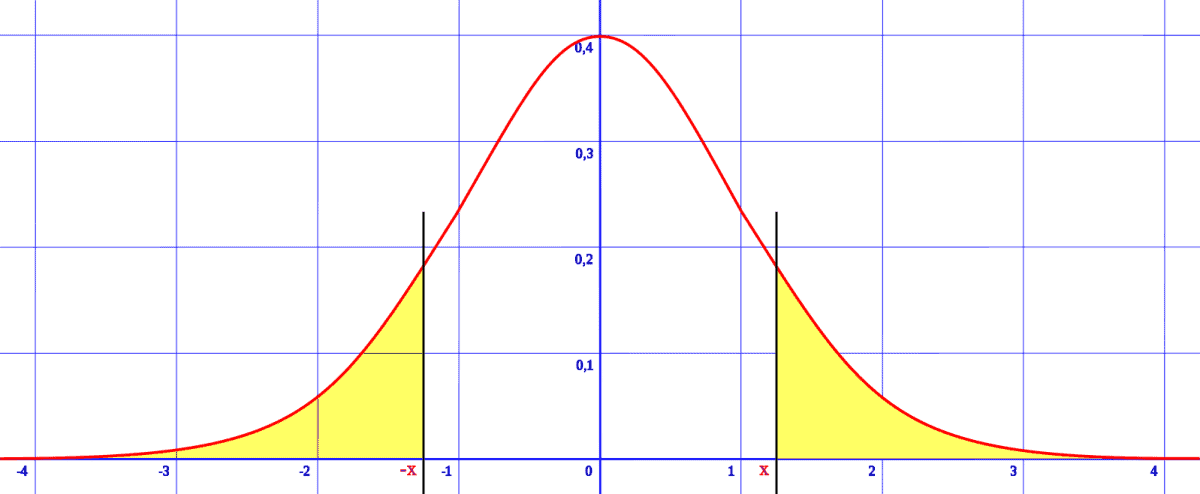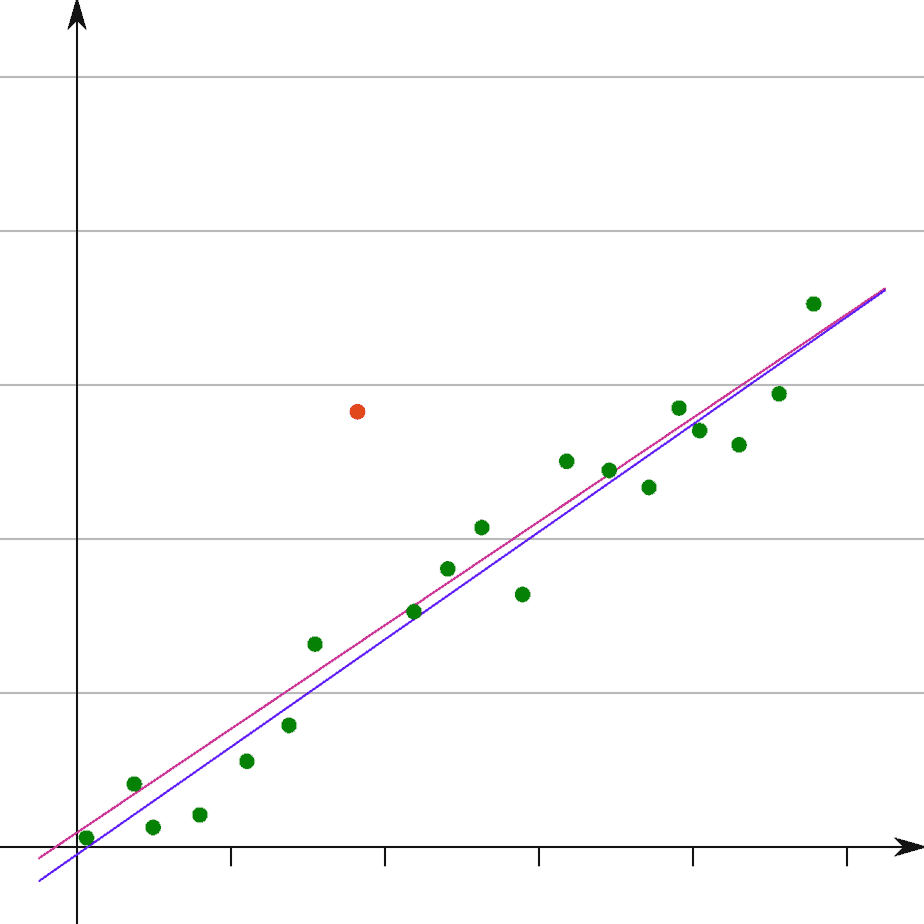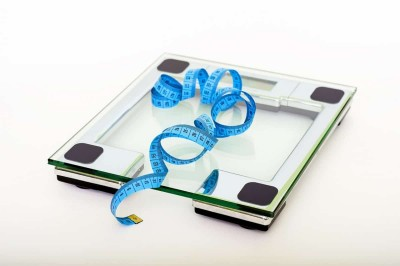# What Affects Standard Deviation? (6 Factors To Consider)

Standard deviation is used in statistics to tell us how “spread out” the data points are.  Having one or more data points far away from the mean indicates a large spread – but there are other factors to consider.

So, what affects standard deviation?  Sample size, mean, and data values affect standard deviation, since they are used to calculate standard deviation.  Removing outliers changes sample size and may change the mean and affect standard deviation.  Multiplication and changing units will also affect standard deviation, but addition will not.

Of course, it is possible by chance that removing an outlier will leave the standard deviation unchanged.  It is important to go through the calculations to see exactly what will happen with the data.

In this article, we’ll talk about the factors that affect standard deviation (and which ones don’t). We’ll also look at some examples to make things clear.

Let’s get started.

What Affects Standard Deviation
What Affects Standard Deviation

(You can also see a video summary version of this article on YouTube!)

## What Affects Standard Deviation?

Standard deviation has the formulaThe formula for the unbiased standard deviation of a sample data set from a population (for standard deviation of the entire population, use N instead of N – 1 in the denominator of the fraction in the radical).

Standard deviation is used in fields from business and finance to medicine and manufacturing.

Some of the things that affect standard deviation include:

• Sample Size – the sample size, N, is used in the calculation of standard deviation and can affect its value.
• Removing Outliers – removing an outlier changes both the sample size (N) and the mean, so it can affect the standard deviation.
• Multiplication – multiplying all data values by a constant value K will affect the standard deviation (scaling it by K).
• Addition – adding (or subtracting) the same value to every data point will change the mean, but it will not change the standard deviation.
• Changing Units – changing units is multiplication by a constant, so it will affect standard deviation (for example, changing from feet to inches means multiplying by 12).
• Mean – the mean is used in the calculation of standard deviation and can affect its value.Alaska is an extreme outlier when it comes to the land area of U.S. states. Texas is also a bit of an outlier. California is above the other 47 states.

Let’s take a look at each of these factors, along with some examples, to see how they affect standard deviation.

### Does Sample Size Affect Standard Deviation?

Sample size does affect the sample standard deviation.  However, it does not affect the population standard deviation.

The sample size, N, appears in the denominator under the radical in the formula for standard deviation.Standard deviation tells us how “spread out” the data points are. Changing the sample size (number of data points) affects the standard deviation.

So, changing the value of N affects the sample standard deviation.  Changing the sample size N also affects the sample mean (but not the population mean).

#### Example 1: Changing N Changes Standard Deviation

For the data set S = {1, 3, 5}, we have the following:

• N = 3 (there are 3 data points)
• Mean = 3 (since (1 + 3 + 5) / 3 = 3)
• Standard Deviation = 2

If we change the sample size by removing the third data point (5), we have:

• S = {1, 3}
• N = 2 (there are 2 data points left)
• Mean = 2 (since (1 + 3) / 2 = 2)
• Standard Deviation = 1.41421 (square root of 2)

So, changing N changed both the mean and standard deviation.

Of course, it is possible by chance that changing the sample size will leave the standard deviation unchanged.

#### Example 2: Changing N Leaves Standard Deviation Unchanged

For the data set S = {1, 2, 2.36604}, we have the following:

• N = 3 (there are 3 data points)
• Mean = 1.78868 (since (1 + 2 + 2.36604) / 3 = 3)
• Standard Deviation = 0.70711

If we change the sample size by removing the third data point (2.36604), we have:

• S = {1, 2}
• N = 2 (there are 2 data points left)
• Mean = 1.5 (since (1 + 2) / 2 = 1.5)
• Standard Deviation = 0.70711

So, changing N lead to a change in the mean, but leaves the standard deviation the same.

### Does Removing An Outlier Affect Standard Deviation?

Removing an outlier affects standard deviation.  In removing an outlier, we are changing the sample size N, the mean, and thus the standard deviation.An outlier is a data point that is far outside of the expected range of values (far higher or lower than other data points).

#### Example: Removing An Outlier Changes Standard Deviation

For the data set S = {1, 3, 98}, we have the following:

• N = 3 (there are 3 data points)
• Mean = 34 (since (1 + 3 + 98) / 3 = 34)
• Standard Deviation = 55.4346

If we change the sample size by removing the third data point (98), we have:

• S = {1, 2}
• N = 2 (there are 2 data points left)
• Mean = 2 (since (1 + 3) / 2 = 2)
• Standard Deviation = 1.41421 (square root of 2)

So, changing N changed both the mean and standard deviation (both in a significant way).

### Does Addition Affect Standard Deviation?

Addition of the same value to every data point does not affect standard deviation.  However, it does affect the mean.

This is because standard deviation measures how spread out the data points are.  Adding the same value to every data point may give us larger values, but they are still spread out in the exact same way (in other words, the distance between data points has not changed at all!)

#### Example: Addition Does Not Change Standard Deviation.

For the data set S = {1, 2, 3}, we have the following:

• N = 3 (there are 3 data points)
• Mean = 2 (since (1 + 2 + 3) / 3 = 2)
• Standard Deviation = 1

If we add the same value of 5 to each data point, we have:

• S = {6, 7, 8}
• N = 3 (there are still 3 data points)
• Mean = 7 (since (6 + 7 + 8) / 3 = 7)
• Standard Deviation = 1

So, adding 5 to all data points changed the mean (an increase of 5), but left the standard deviation unchanged (it is still 1).

### Does Multiplication Affect Standard Deviation?

Multiplication affects standard deviation by a scaling factor.  If we multiply every data point by a constant K, then the standard deviation is multiplied by the same factor K.

In fact, the mean is also scaled by the same factor K.

#### Example: Multiplication Scales Standard Deviation By A Factor Of K

For the data set S = {1, 2, 3}, we have the following:

• N = 3 (there are 3 data points)
• Mean = 2 (since (1 + 2 + 3) / 3 = 2)
• Standard Deviation = 1

If we use multiplication by a factor of K = 4 on every point in the data set, we have:

• S  = {4, 8, 12}
• N = 3 (there are still 3 data points)
• Mean = 8 (since (4 + 8 + 12) / 3 = 8)
• Standard Deviation = 4

So, multiplying by K = 4 also multiplied the mean by 4 (it went from 2 to 8) and multiplied standard deviation by 4 (it went from 1 to 4).

### Does Changing Units Affect Standard Deviation?

Changing units affects standard deviation.  Any change in units will involve multiplication by a constant K, so the standard deviation (and the mean) will also be scaled by K.Changing units of measurement (like inches instead of feet for height) will change standard deviation accordingly.

#### Example: Changing Units Changes Standard Deviation

For the data set S = {1, 2, 3} (units in feet), we have the following:

• N = 3 (there are 3 data points)
• Mean = 2 feet (since (1 + 2 + 3) / 3 = 2)
• Standard Deviation = 1 foot

If we want to convert units from feet to inches, we use multiplication by a factor of K = 12 on every point in the data set, we have:

• S  = {12, 24, 36}
• N = 3 (there are still 3 data points)
• Mean = 24 (since (12 + 24 + 36) / 3 = 24)
• Standard Deviation = 12

So, multiplying by K = 12 also multiplied the mean by 12 (it went from 2 to 24) and multiplied standard deviation by 12 (it went from 1 to 12).

### Does Mean Affect Standard Deviation?

Mean affects standard deviation.  To calculate standard deviation, we add up the squared differences of every data point and the mean.

However, it can happen by chance that a different mean will lead to the same standard deviation (for example, when we add the same value to every data point).

This is because standard deviation measures how far each data point is from the mean.  So, the data set {1, 3, 5} has the same standard deviation as the set {2, 4, 6} (all we did was add 1 to each data point in the first set to get the second set).

See the example from earlier (adding 5 to every data point in the set {1, 2, 3}): the mean changes, but the standard deviation does not.

## Conclusion

Now you know what affects standard deviation and what to consider about outliers and sample size.

You can learn about the units for standard deviation here.

You can learn about the difference between standard deviation and standard error here.

You might also be interested to learn more about variance in my article here.

This article I wrote will reveal what standard deviation can tell us about a data set.

I hope you found this article helpful.  If so, please share it with someone who can use the information.

~Jonathon